# Quiz 4: Linear Programming: Modeling Examples

Business

The sensitivity report of the problem is shown below: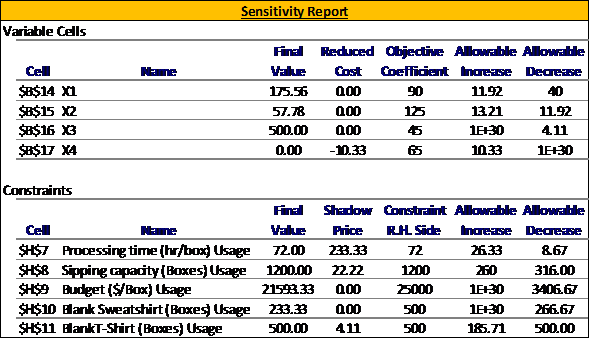The problem can't be solved just by using the sensitivity report. This is because, the modification requested consist of changing the coefficient of the constraint for which sensitivity report is not effective in calculating the change. Step-1: Solve the problem with new data using solver as shown below::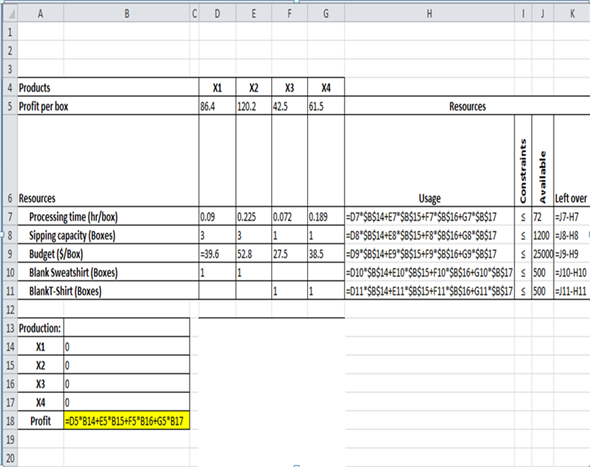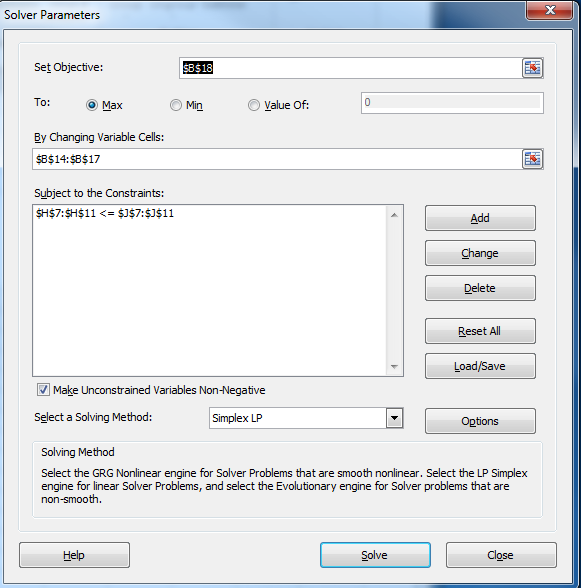Step-2: Click on solve: The new solution generated by the solver is shown below: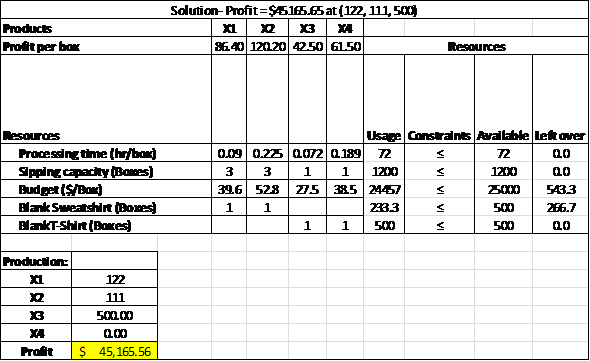The profit with new reduced processing time is \$45,165 which is less than the profit as calculate in the reference problem i.e. \$45,522. Thus, it is advised not to undertake this alternative. Shadow price is the change in the optimal value per unit change in the right hand side of the constraint up to the sensitivity range limit. Thus, according to the definition if the company decides to acquire extra T-shirts, the company will earn an additional \$4.11 for each extra T-shirt it acquires above 500, up to the sensitivity range limit i.e. 685.71. But, the additional information says that the profit consists of fixed costs plus variable costs. Under this assumption,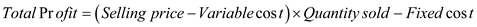Thus one can see there is a nonlinear relationship and thus with increase in quantity the profit will rise non-linearly i.e. the average profit per unit will increase. Thus, if the company decides to acquire extra T-shirts, the company will not earn an additional \$4.11 for each extra T-shirt it acquires above 500, up to the sensitivity range limit i.e. 685.71. If the company decides to produce equal number of each type of t-shirts the company is required to add the following additional constraint in the linear programming model: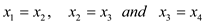Add the additional constraint (shown in red color) in the solve sheet, as shown below, and click on solve: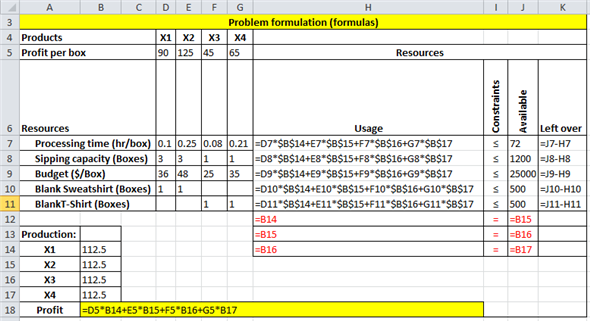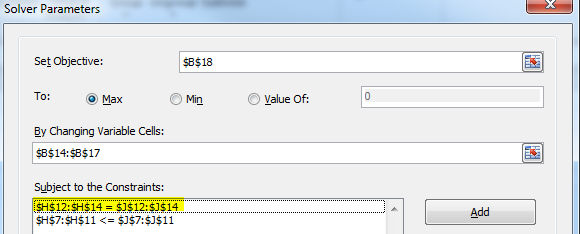The result of the solver is shown below: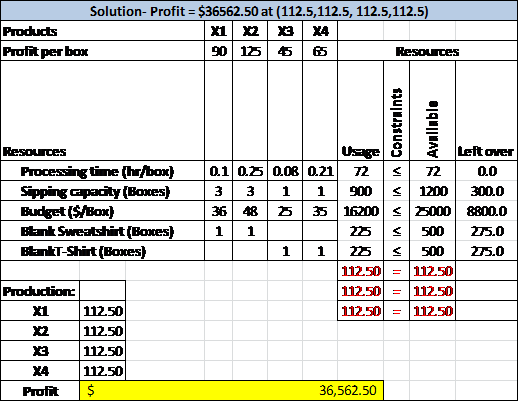The profit is \$36562.50.

Company S is a garden tool manufacturing company. The company manufactures four garden tools made up of steel with wooden handle. The process of manufacturing includes 2 stages. Stage 1 includes two operations - stamping and drilling process. The stage 2 involves 3 operations - Assembly, finishing and packaging. Formulate the linear programming model and consider the following information of decision variable:Objective function is to minimize the cost: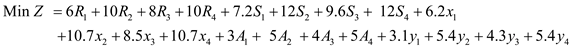Constraints are subjected to: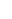Non-negativity constraints are: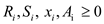Prepare an excel spreadsheet for the company S manufacturing four tools, as shown below: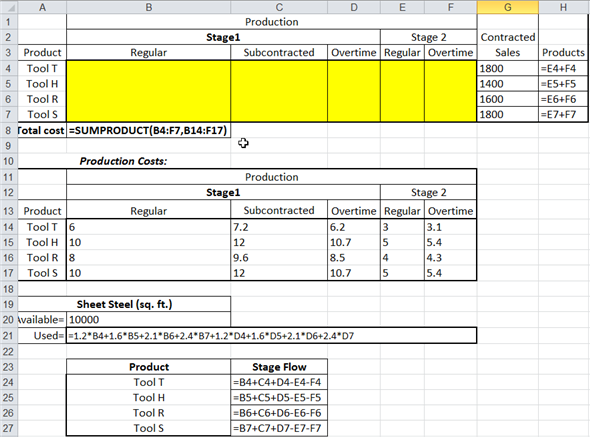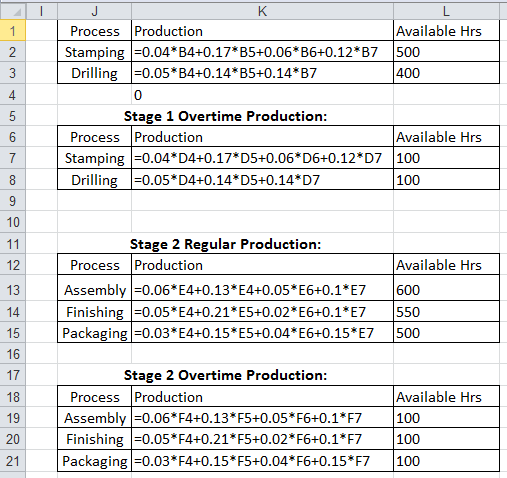The spreadsheet obtained is shown below: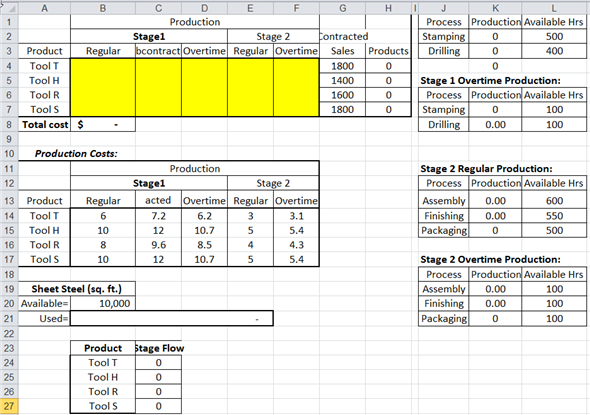Select " Solver " from the " Data " menu bar, as shown below:A pop-up window would appear after clicking on " Solver ". Fill in the objective function and constraints as shown below: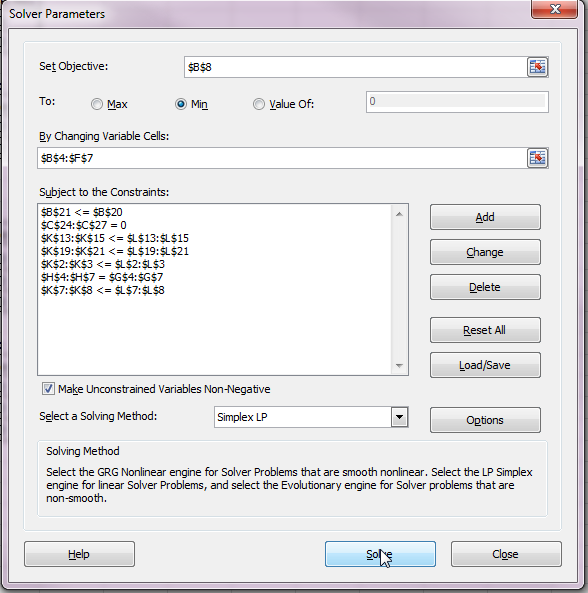Click on " Solve " option. Then the following pop-up would appear: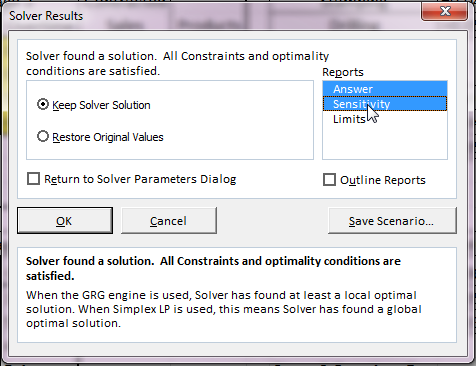Select "Answer" and "Sensitivity" option present under "Reports". Then, click on " OK " option. It would give the following results: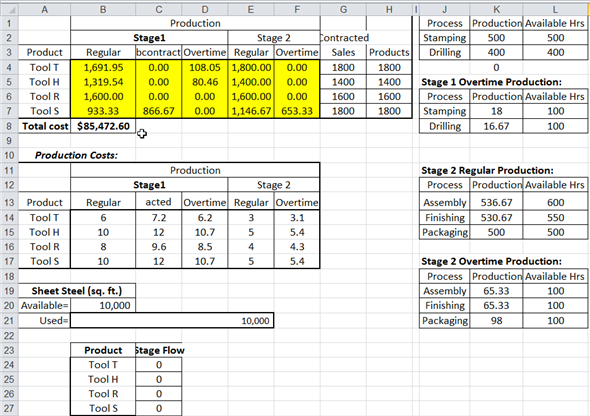Hence, the minimum cost of manufacturing tools in regular time, subcontracted, and overtime is \$85,472.60 Observe the sensitivity report as shown below: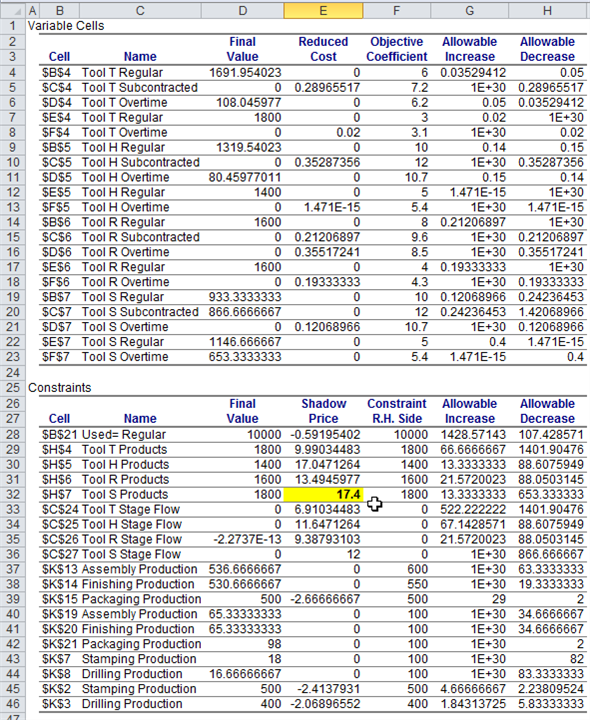From the above figure, it is clear that the shadow price of tool S is \$17.4. It indicates that Tool H appears to be the most critical resource to increase profit and production process.

This is a case of integer programming because the sheets can't take fractional values. Follow the following steps to solve the model: Step-1: Decide the decision variables: Let,Step-2: Formulate the objective function; the objective is to minimize the cost. Writing this statement in mathematical form as shown below: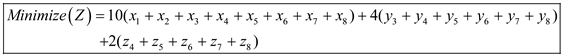Step-3: Formulate the constraints, as shown below: Note-1 : Due to lead time of 1 week, the sheets given to the laundry at the end of week-1 will satisfy the demand of week-3. Note-2: Due to lead time of 2 week, the sheets given to the friends for washing at the end of week-1 will satisfy the demand of week-4. Note-3: There will be 20% return for each sheet washed. Subject to:Now, create constraints for the number of sheets that can be given for washing:Solve the model using Excel solver as shown below: Step-1: Open Excel and create the model as shown below: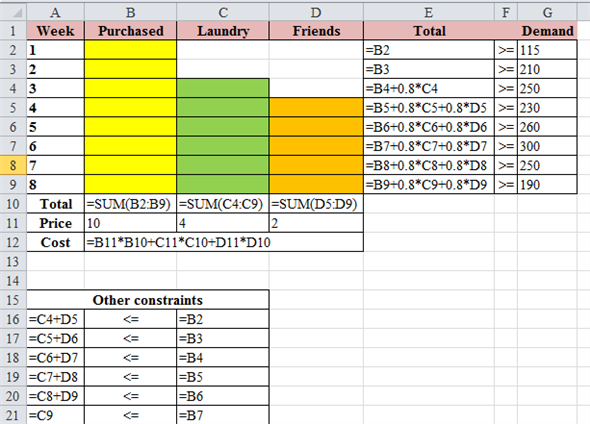Step-2: Go to Data, add the constraints requisite values and click 'Solver' as shown below: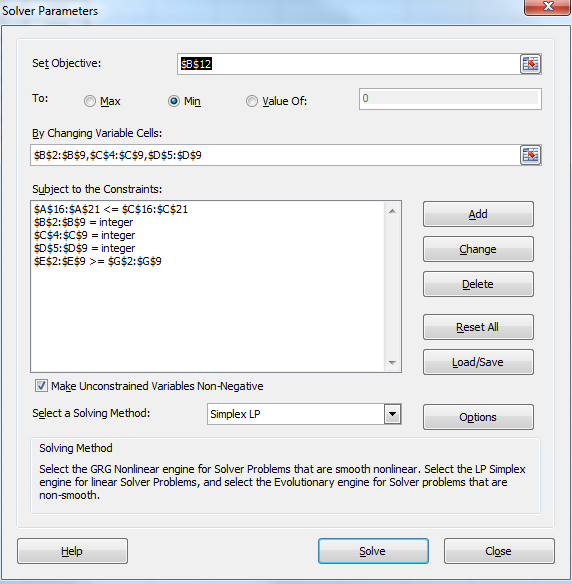Step-3: Go to the options menu and decrease the constraint precision to the value shown in the screenshot shown below: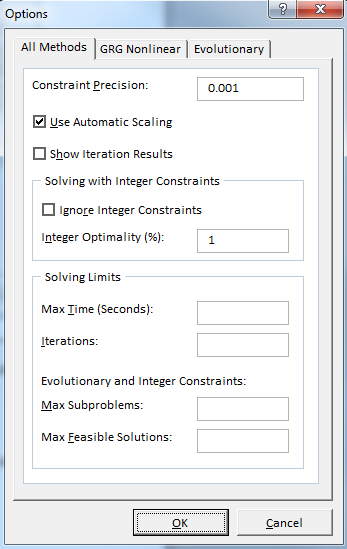Step-4: Click on "OK" and then on "Solve" to generate the results: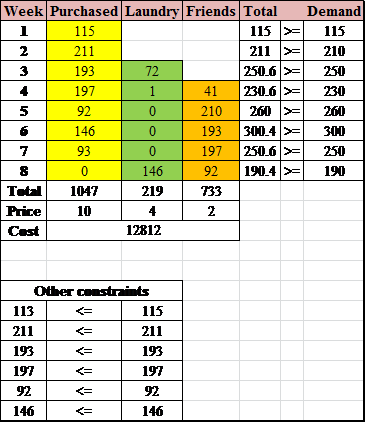The plan for purchase is shown in the table.

There is no answer for this question

There is no answer for this question